# Fraction calculator

The calculator performs basic and advanced operations with fractions, expressions with fractions combined with integers, decimals, and mixed numbers. It also shows detailed step-by-step information about the fraction calculation procedure. Solve problems with two, three, or more fractions and numbers in one expression.

## Result:

### 33/4 * 22/5 = 9/1 = 9

Spelled result in words is nine.

### How do you solve fractions step by step?

1. Conversion a mixed number 3 3/4 to a improper fraction: 3 3/4 = 3 3/4 = 3 · 4 + 3/4 = 12 + 3/4 = 15/4

To find a new numerator:
a) Multiply the whole number 3 by the denominator 4. Whole number 3 equally 3 * 4/4 = 12/4
b) Add the answer from previous step 12 to the numerator 3. New numerator is 12 + 3 = 15
c) Write a previous answer (new numerator 15) over the denominator 4.

Three and three quarters is fifteen quarters
2. Conversion a mixed number 2 2/5 to a improper fraction: 2 2/5 = 2 2/5 = 2 · 5 + 2/5 = 10 + 2/5 = 12/5

To find a new numerator:
a) Multiply the whole number 2 by the denominator 5. Whole number 2 equally 2 * 5/5 = 10/5
b) Add the answer from previous step 10 to the numerator 2. New numerator is 10 + 2 = 12
c) Write a previous answer (new numerator 12) over the denominator 5.

Two and two fifths is twelve fifths
3. Multiple: 15/4 * 12/5 = 15 · 12/4 · 5 = 180/20 = 9 · 20/1 · 20 = 9
Multiply both numerators and denominators. Result fraction keep to lowest possible denominator GCD(180, 20) = 20. In the following intermediate step, cancel by a common factor of 20 gives 9/1.
In other words - fifteen quarters multiplied by twelve fifths = nine.

#### Rules for expressions with fractions:

Fractions - use the slash “/” between the numerator and denominator, i.e., for five-hundredths, enter 5/100. If you are using mixed numbers, be sure to leave a single space between the whole and fraction part.
The slash separates the numerator (number above a fraction line) and denominator (number below).

Mixed numerals (mixed fractions or mixed numbers) write as non-zero integer separated by one space and fraction i.e., 1 2/3 (having the same sign). An example of a negative mixed fraction: -5 1/2.
Because slash is both signs for fraction line and division, we recommended use colon (:) as the operator of division fractions i.e., 1/2 : 3.

Decimals (decimal numbers) enter with a decimal point . and they are automatically converted to fractions - i.e. 1.45.

The colon : and slash / is the symbol of division. Can be used to divide mixed numbers 1 2/3 : 4 3/8 or can be used for write complex fractions i.e. 1/2 : 1/3.
An asterisk * or × is the symbol for multiplication.
Plus + is addition, minus sign - is subtraction and ()[] is mathematical parentheses.
The exponentiation/power symbol is ^ - for example: (7/8-4/5)^2 = (7/8-4/5)2

#### Examples:

subtracting fractions: 2/3 - 1/2
multiplying fractions: 7/8 * 3/9
dividing Fractions: 1/2 : 3/4
exponentiation of fraction: 3/5^3
fractional exponents: 16 ^ 1/2
adding fractions and mixed numbers: 8/5 + 6 2/7
dividing integer and fraction: 5 ÷ 1/2
complex fractions: 5/8 : 2 2/3
decimal to fraction: 0.625
Fraction to Decimal: 1/4
Fraction to Percent: 1/8 %
comparing fractions: 1/4 2/3
multiplying a fraction by a whole number: 6 * 3/4
square root of a fraction: sqrt(1/16)
reducing or simplifying the fraction (simplification) - dividing the numerator and denominator of a fraction by the same non-zero number - equivalent fraction: 4/22
expression with brackets: 1/3 * (1/2 - 3 3/8)
compound fraction: 3/4 of 5/7
fractions multiple: 2/3 of 3/5
divide to find the quotient: 3/5 ÷ 2/3

The calculator follows well-known rules for order of operations. The most common mnemonics for remembering this order of operations are:
PEMDAS - Parentheses, Exponents, Multiplication, Division, Addition, Subtraction.
BEDMAS - Brackets, Exponents, Division, Multiplication, Addition, Subtraction
BODMAS - Brackets, Of or Order, Division, Multiplication, Addition, Subtraction.
GEMDAS - Grouping Symbols - brackets (){}, Exponents, Multiplication, Division, Addition, Subtraction.
Be careful, always do multiplication and division before addition and subtraction. Some operators (+ and -) and (* and /) has the same priority and then must evaluate from left to right.

## Fractions in word problems:

• Farmer PeterFarmer Peter paints 12 chicken coop. He started painting this day morning. Now he only has 1/4 of the chicken coop left to paint this afternoon. How many chicken coops did farmer Peter paint this morning?
• Two divided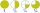Two divided by nine-tenths.
• In bucket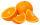In bucket of 87 oranges 1/3 are spoiled ones and the remaining are eaten by the member of a family. How many oranges are eaten by the member of family?
• Twelve eggs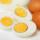A dozen eggs cost 80 cents . how much will 3½ dozen eggs cost?
• Bananas plantation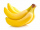Ashok Rao planted bananas on 2/7 th of his field on his 21 acers. What is the area of the bananas plantation?
• Diamonds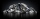If simva had 8 diamond and cuts them each into 1/3 pieces, then how many pieces does she have?
• Jim collected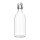Jim collected 5 bins of glass bottles to recycle. Lucia collected 3 1/5 times as many bins as Jim. How many bins of bottles did Lucia collect?
• Lunch timeIn a cafeteria, 3/10 of the students are eating salads, and 3/5 are eating sandwiches. There are 30 students in the cafeteria. How many students are eating lunches other than salads or sandwiches?
• Mario 2Mario is preparing a dinner for his friends. He made 3 bowls of spaghetti sauce. If each bowl holds 3/4 quart, how much sauce did Mario make?
• Evaluate expressionEvaluate expression using BODMAS rule: 1 1/4+1 1/5÷3/5-5/8
• Pupils 7There are 40 pupils in a certain class. 3/5 of the class are boys. How many are girls?
• Length 7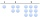Length equals 7/6 inch in width equals 7/9 inches the area is?
• Long and wideWhat is the area of a rectangle that is 1 1/4 meters long and 3/4​ of a meter wide